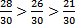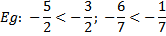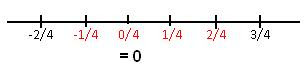## 20 January 2014

Comparison of Rational Numbers

• While comparing positive rational numbers with the same denominator, the number with the greatest numerator is the largest.  It is easy to compare these numbers if their denominators are the same. Eg:• A positive rational number is always greater than a negative rational number.
• While comparing negative rational numbers with the same denominator, compare their numerators ignoring the minus sign. The number with the greatest numerator is the smallest.
•• Positive rational numbers lie to the right of 0, while negative rational numbers lie to the left of 0 on the number line.• To compare rational numbers with different denominators, convert them into equivalent rational numbers with the same denominator, which is equal to the LCM of their denominators.  You can find infinite rational numbers between any two given rational numbers.

#Operations on Rational Numbers
• The denominator of the sum or difference of two rational numbers with the same denominator is the same as the common denominator of the given numbers.
• The numerator of the sum of two rational numbers with the same denominator is the sum of the numerators of the given numbers with their correct sign.
• The numerator of the difference of two rational numbers with the same denominator is the difference between the numerators of the given numbers with their correct sign.
• To add or subtract rational numbers with different denominators, we convert them into equivalent rational numbers having common denominator equal to the LCM of the denominators of the given numbers.
• Two rational numbers whose sum is zero are called additive inverse of each other.
• The numerator and denominator of the product of two rational numbers are equal to the product of their individual numerators and denominators.
• Two rational numbers whose product is 1 are called reciprocals of each other.
• A rational number and its reciprocal always have the same sign.
• To divide one rational number by a second rational number, we actually multiply the first number by the reciprocal of the second number.

 With our free PDF notes you can get success in any competitive or entrance exams like CTET,  KVS, NET, CAT, MAT, CMAT, SSC, B.ED, IBPS Recruitment, IAS, CSAT, State Civil Services Exams, UPTET, PSTET, HTET & many more. It also provides NCERT solutions, CBSE, NTSE, Olympiad study material, Indian General Knowledge, English, Hindi, Mathematics, Current affairs, Science, S.ST, model test papers, important Questions and Answers asked in CBSE examinations.